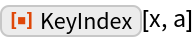Function Repository Resource:

KeyIndex

Create an association indexed by a given key from a list of associations

Contributed by: Richard Hennigan (Wolfram Research)
 ResourceFunction["KeyIndex"][{a1,a2,…},key] gives an Association that has keys ai[key] and values ai with key dropped. ResourceFunction["KeyIndex"][key] represents an operator form of ResourceFunction["KeyIndex"] that can be applied to an expression.

Details and Options

Each of the ai can be either an Association or a List of rules.
In ResourceFunction["KeyIndex"][expr,key] for non-atomic expr, the expr can have any head, not just List.
ResourceFunction["KeyIndex"][Dataset[],] is also supported when the dataset is formed from a list of associations.
ResourceFunction["KeyIndex"][assoc,] is equivalent to ResourceFunction["KeyIndex"][Values[assoc],] when assoc is an Association.
ResourceFunction["KeyIndex"] has the following options:
 "DropKey" True whether to drop key from each of the ai MergingFunction Automatic determine how ai and aj should be combined when ai[key]⩵aj[key] MissingBehavior Automatic how to handle ai that do not have key
The values for MissingBehavior determine how to handle ai that do not have the given key:
 Automatic discard ai that lack the given key None include ai under the key Missing["KeyAbsent",key] in the result

Examples

Basic Examples

Index a list of associations by a given key:

 In:=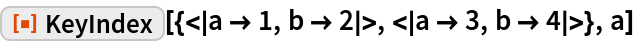Out=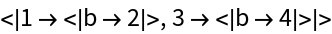Index lists of rules:

 In:=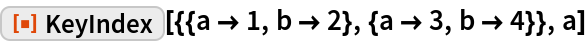Out=Use a combination of lists and associations:

 In:=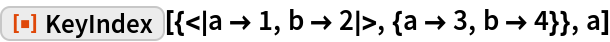Out=Use the operator form:

 In:=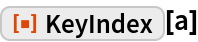Out=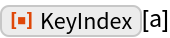In:=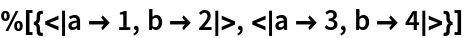Out=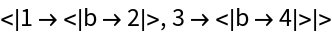Index a Dataset by a given key:

 In:=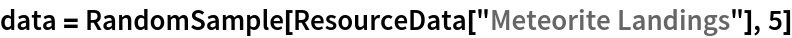Out=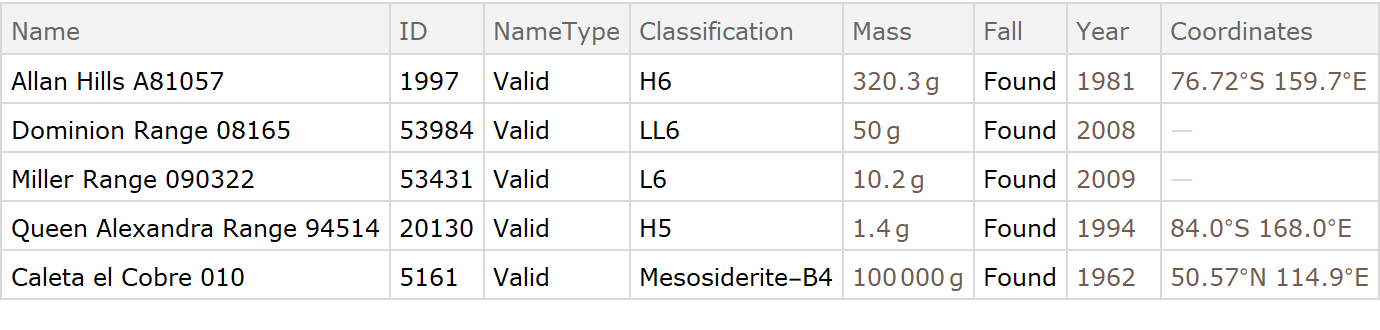In:=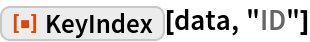Out=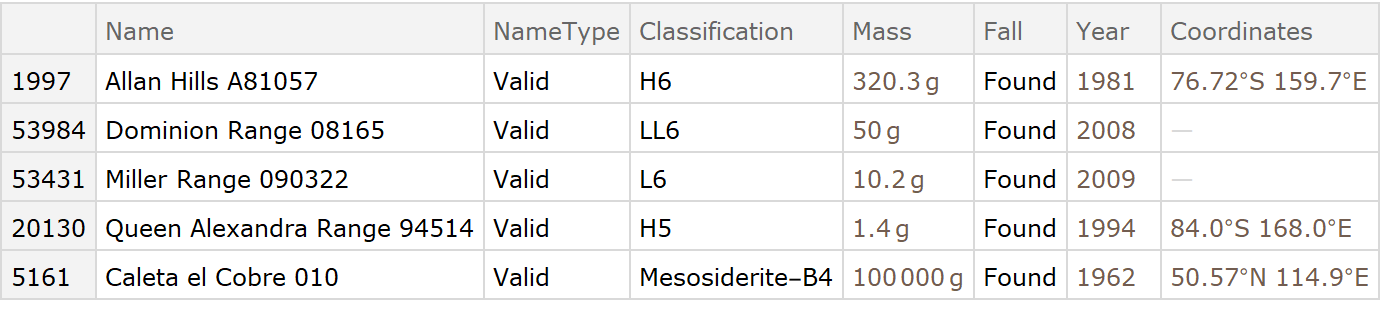Scope

The head of expr doesn’t necessarily have to be List:

 In:=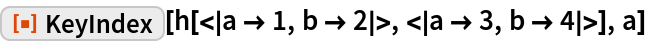Out=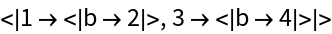If expr is an Association, KeyIndex uses Values[expr]:

 In:=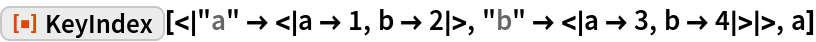Out=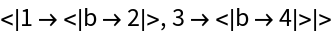Options

DropKey

Drop the given key from each of the resulting associations:

 In:=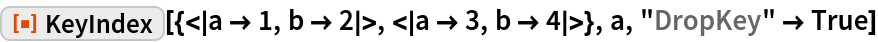Out=Leave the given key in the results:

 In:=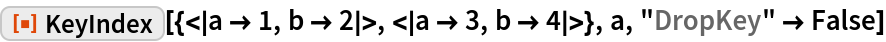Out=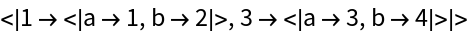MergingFunction

By default, only the first value will be used when there is a collision:

 In:=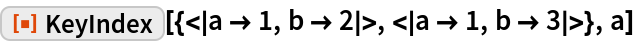Out=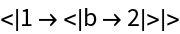Specify how collisions should be handled:

 In:=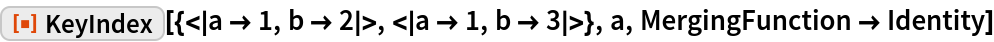Out=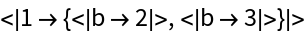Combine values with Merge:

 In:=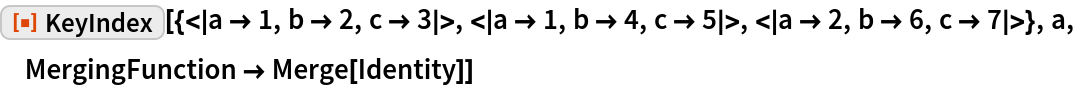Out=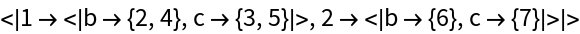MissingBehavior

The default behavior of KeyIndex is to discard associations that lack the given key in the result:

 In:=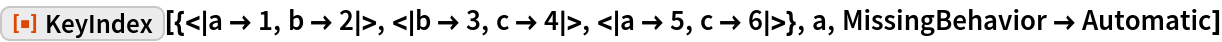Out=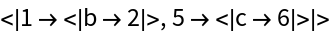Don’t apply special rules if the key is missing:

 In:=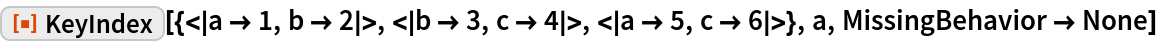Out=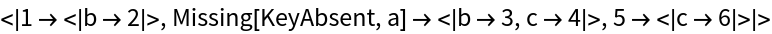Applications

Create a new Dataset from an existing one that is indexed by a particular key:

 In:=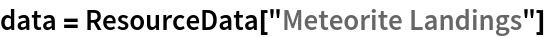Out=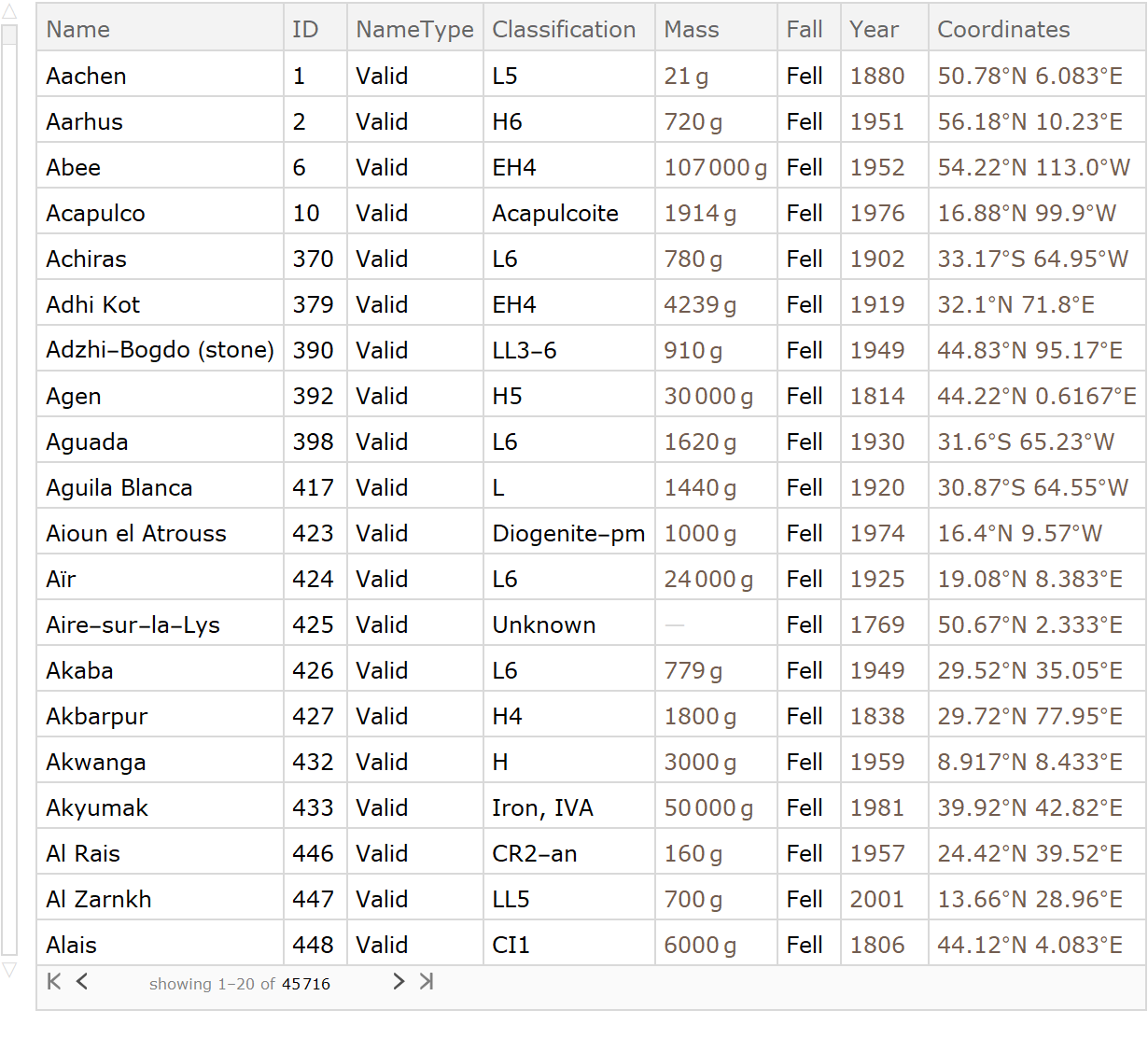In:=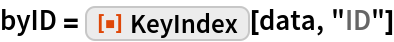Out=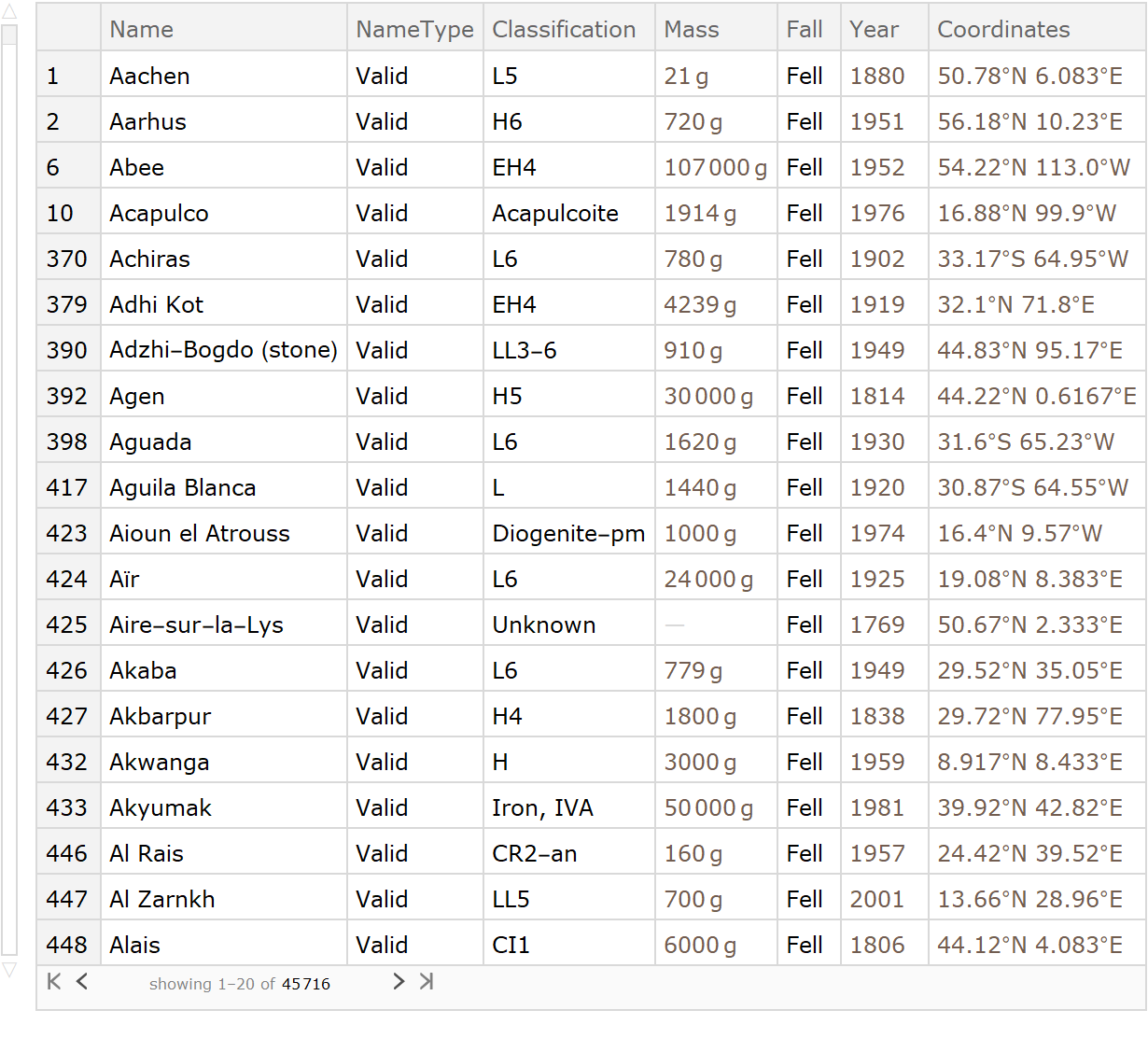Look up data using the new index:

 In:=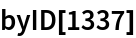Out=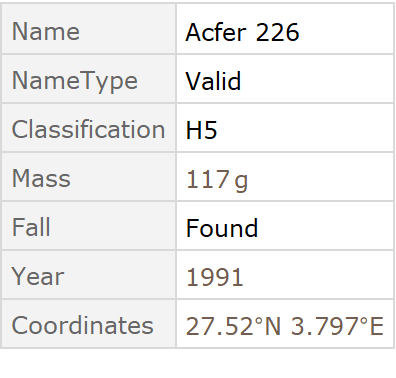Create groups by a given key:

 In:=Out=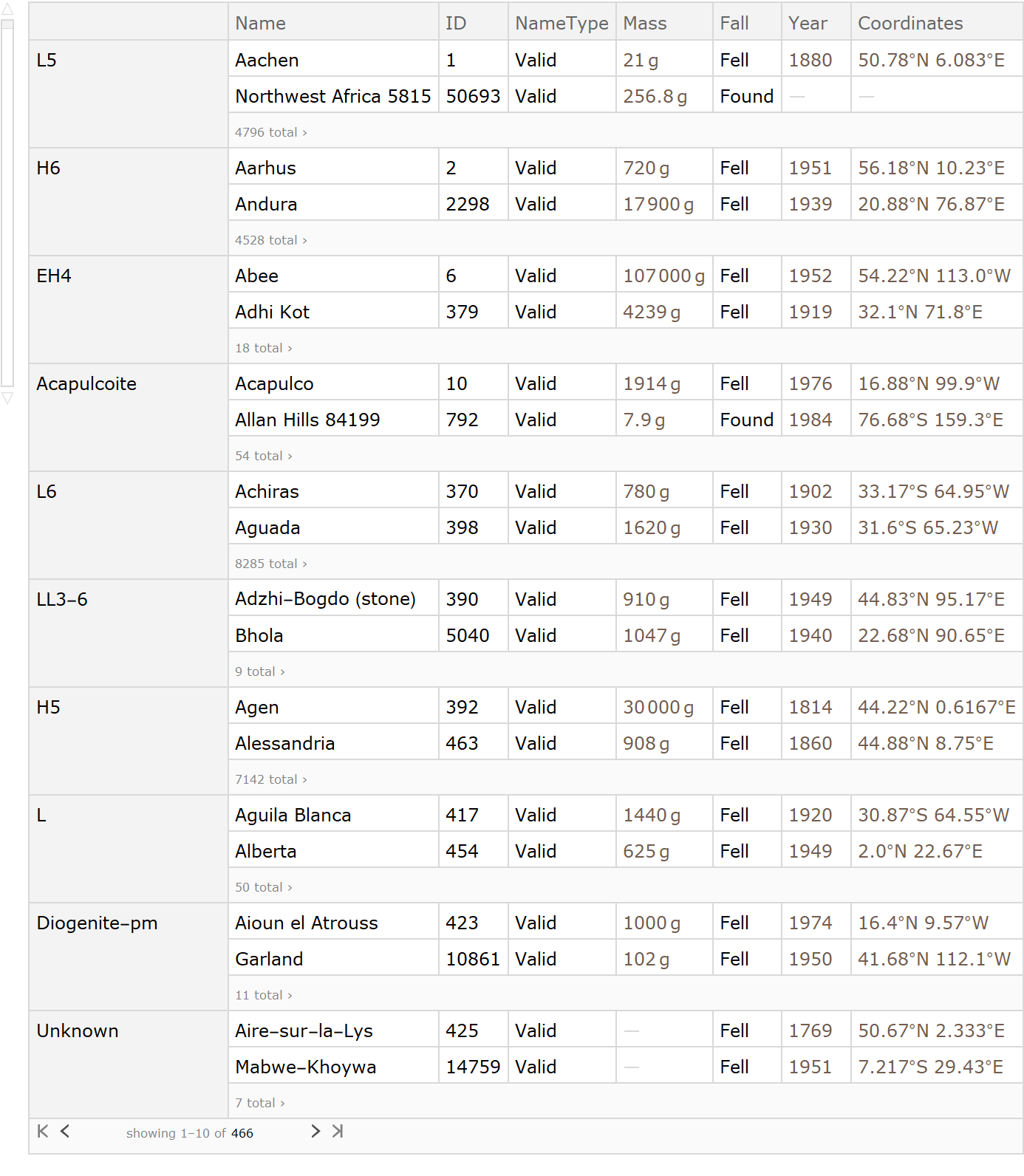Get the set matching a given classification:

 In:=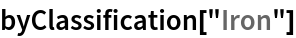Out=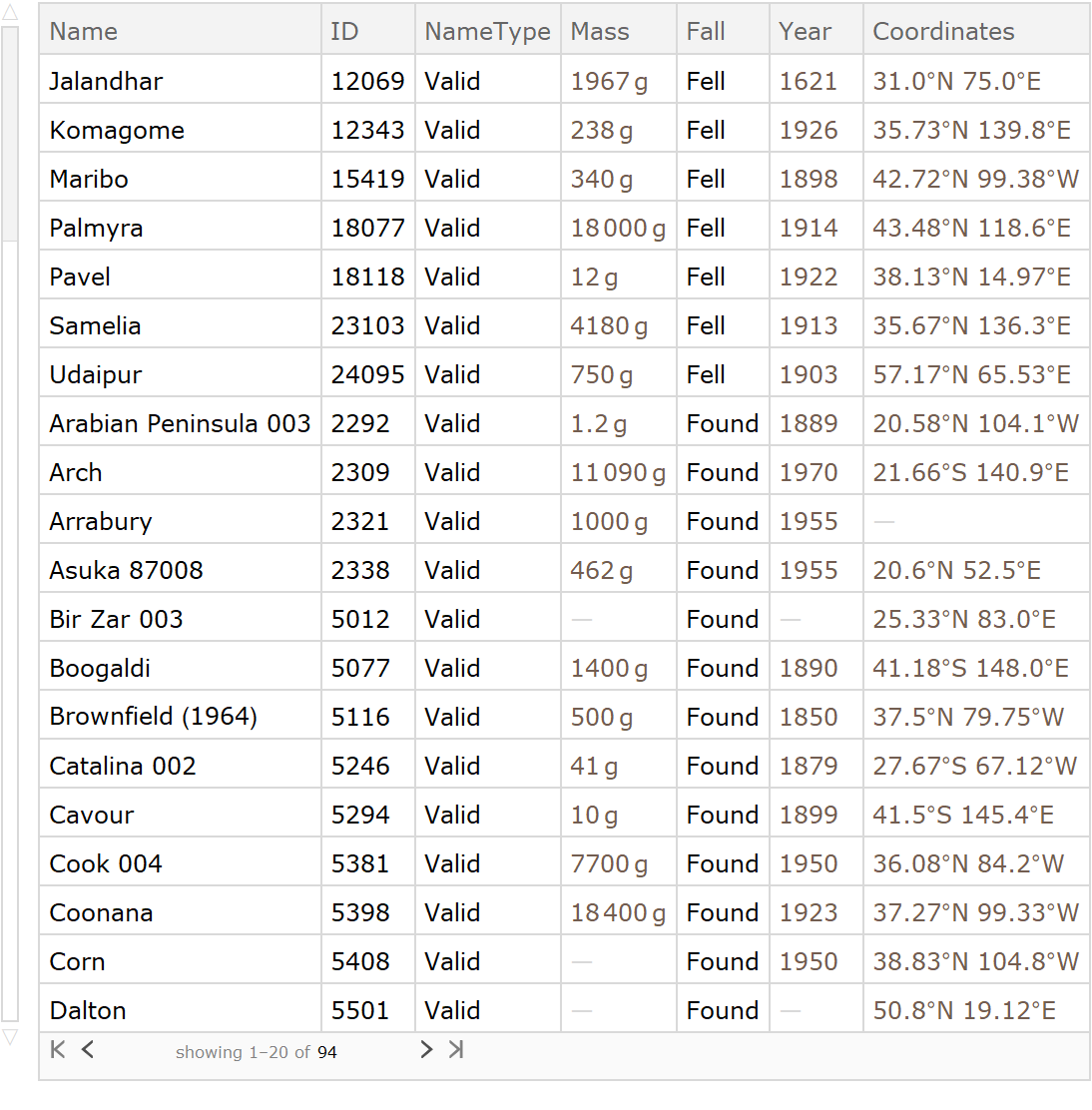Properties and Relations

KeyIndex[expr,key] is similar to GroupBy[expr,Key[key]]:

 In:=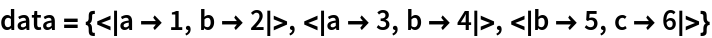Out=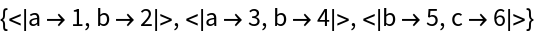In:=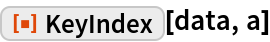Out=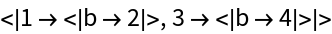In:=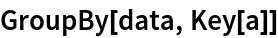Out=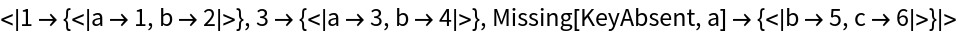Similar behavior can be obtained by setting options:

 In:=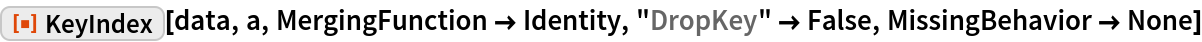Out=The default behavior of KeyIndex does additional post-processing of the data:

 In:=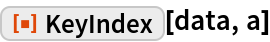Out=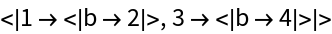In:=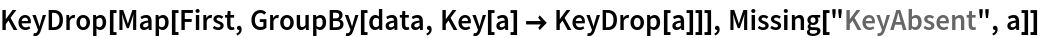Out=Possible Issues

Each item must be an Association or a List of rules:

 In:=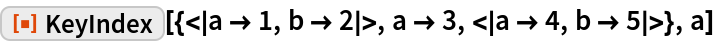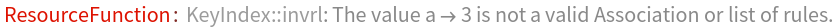Out=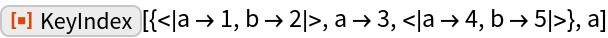In:=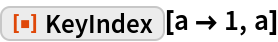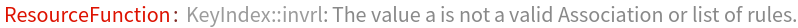Out=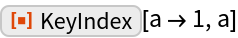In:=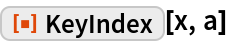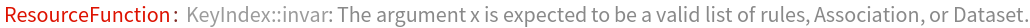Out=